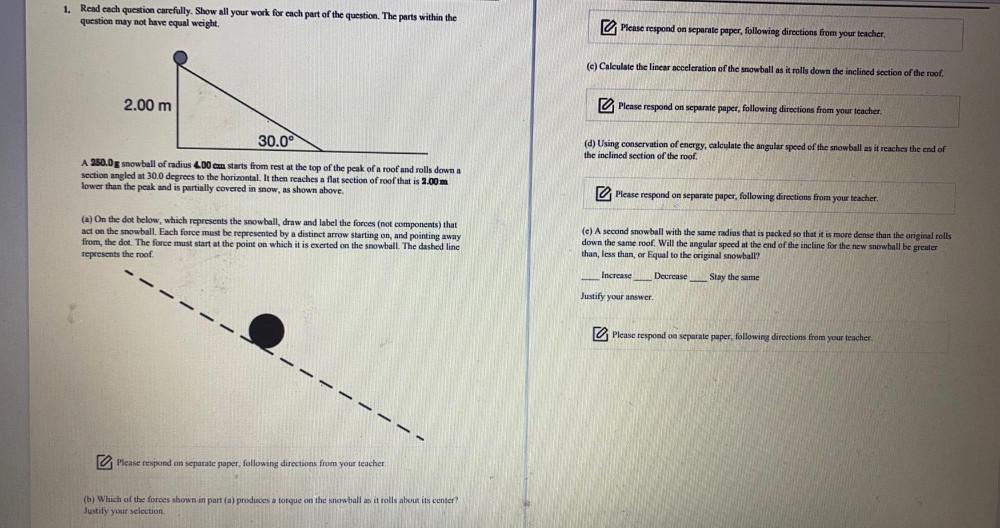Question:

# Read each question carefully, Show all your work for each part of the question. The parts within the question may not have equal weight. A 250.0 g snowball of radius 4.00 cm starts from rest at the toRead each question carefully, Show all your work for each part of the question. The parts within the question may not have equal weight. A 250.0 g snowball of radius 4.00 cm starts from rest at the top of the peak of a roof and rolls down a section angled at 30.0 degrees to the horizontal. It then reaches a flat section of roof that is 2.00m lower than the peak and is partially covered in snow, as shown above. (a) On the dot below, which represents the snowball, draw and label the forces (not components) that act on the snowball. Each force must be represented by a distinct arrow starting on, and pointing away from, the dot. The force must start at the point on which it is exerted on the snowball. The dashed line represents the roof. (b) Which of the forces shown in part (a) produces a torque on the snowball as it rolls about its center? (c) Calculate the linear acceleration of the snowball as it rolls down the inclined section of the roof. (d) Using conservation of energy, calculate the angular speed of the snowball as it reaches the end of the inclined section of the roof. (e) A second snowball with the same radius that is packed so that it is more dense than the original rolls down the same roof. Will the angular speed at the end of the incline for the new snowball be greater than, less than, or Equal to the original snowball? (1)Increase (2)Decrease (3)Stay the same.